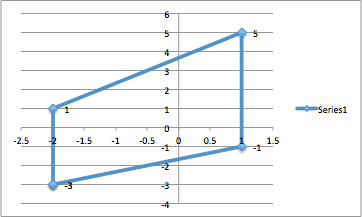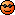# Green's Theorem homework problem

## Homework Statement

Let C be the closed, piecewise smooth curve formed by traveling in straight lines between the points (-2,1), (-2,-3), (1,-1), (1,5) and back to (-2,1), in that order. Use Green's Theorem to evaluate the integral ∫(2xy)dx+(xy2)dy.

## Homework Equations

Green's Theorem:

∫Pdx+Qdy = ∫∫(∂Q/∂x-∂P/∂y)dxdy

## The Attempt at a Solution

I first found ∂Q/∂x to be 2y2 and ∂P/∂y to be 2x.

I am struggling to find bounds that are easy to use for the double integral. I tried keeping x fixed and integrating from the y = (2/3)x-5/3 to y = (4/3)x+11/3. But that got messy quickly.

Then I tried keeping y fixed and integrating from x = (3/2)y+(5/2) and x=(3/4)y-11/4. I forced my way through that one, but there has got to be a simpler way. Furthermore, I got a wrong answer that way anyway, but I don't know if it's because I'm setting the integral up incorrectly or if I made an error.

Thanks and sorry if the formatting looks weird I've never used this site before.

SteamKing
Staff Emeritus
Homework Helper
Guess what? We don't know what you did wrong, either ... unless you post your calculations.

Guess what? We don't know what you did wrong, either ... unless you post your calculations.

Thanks.

Sorry I should have been more clear when posting this. I'm not really interested in the fact what I did was wrong in the end if technically it should have worked and I just dropped a negative or something somewhere along the way.

If you think what I did was wrong because my bounds were incorrect I can type up my my work.

But maybe it will be helpful to see my integral, so here is what I wrote down:

∫∫(y2-2x)dxdy
With x bounds as I wrote previous and y bounded between -3 and 5.

I just want to know if there's an easier way to do this problem, because it was time consuming. I'm still learning so I often don't see shortcuts.

If not, then cool I set up the problem correctly and just made a mistake along the way. I can go back and see where I went wrong on my own :)

Last edited:
SteamKing
Staff Emeritus
Homework Helper
I think you have to make a sketch of the region as described by the list of points given in the OP. Based on my mental sketch, the region is not a square or rectangle, but some sort of rhomboid.

This is a curious exercise involving the use of the Green's Theorem IMO. In my experience, Green's Theorem is used to convert a double integral into a line integral which can be evaluated by traversing the boundary of the region specified. The line integrals can usually be parameterized so that their evaluation is relatively simple.

LCKurtz
Homework Helper
Gold Member

## Homework Statement

Let C be the closed, piecewise smooth curve formed by traveling in straight lines between the points (-2,1), (-2,-3), (1,-1), (1,5) and back to (-2,1), in that order. Use Green's Theorem to evaluate the integral ∫(2xy)dx+(xy2)dy.

## Homework Equations

Green's Theorem:

∫Pdx+Qdy = ∫∫(∂Q/∂x-∂P/∂y)dxdy

## The Attempt at a Solution

I first found ∂Q/∂x to be 2y2 and ∂P/∂y to be 2x.

Recheck ##Q_x##.

I am struggling to find bounds that are easy to use for the double integral. I tried keeping x fixed and integrating from the y = (2/3)x-5/3 to y = (4/3)x+11/3. But that got messy quickly.

And ##x## from ##-2## to ##1##? That would be a correct setup.

Then I tried keeping y fixed and integrating from x = (3/2)y+(5/2) and x=(3/4)y-11/4. I forced my way through that one, but there has got to be a simpler way. Furthermore, I got a wrong answer that way anyway, but I don't know if it's because I'm setting the integral up incorrectly or if I made an error.

That is an incorrect setup for a ##dxdy## integral. You would have to break it up onto three parts as you will see if you draw the region.

I think you have to make a sketch of the region as described by the list of points given in the OP. Based on my mental sketch, the region is not a square or rectangle, but some sort of rhomboid.

This is a curious exercise involving the use of the Green's Theorem IMO. In my experience, Green's Theorem is used to convert a double integral into a line integral which can be evaluated by traversing the boundary of the region specified. The line integrals can usually be parameterized so that their evaluation is relatively simple.

Wasn't sure of the best way to do this, but here you go :)Recheck ##Q_x##.

And ##x## from ##-2## to ##1##? That would be a correct setup.

That is an incorrect setup for a ##dxdy## integral. You would have to break it up onto three parts as you will see if you draw the region.

Oops I copied my work down incorrectly - ∫∫(2xy-2x)dxdy. I actually did integrate this function.

But I'm confused about what you're trying to say about my bounds. If I integrated from -2 to 1 in the x direction and -3 to 5 in the y direction, I would get a rectangle, wouldn't I? And this is not a rectangle.

And could you explain how my set up is incorrect (where did I go wrong?) and what the three parts of my integral would be? Thank you!

SteamKing
Staff Emeritus
Homework Helper
Wasn't sure of the best way to do this, but here you go :)

View attachment 76684

Oops I copied my work down incorrectly - ∫∫(2xy-2x)dxdy. I actually did integrate this function.

But I'm confused about what you're trying to say about my bounds. If I integrated from -2 to 1 in the x direction and -3 to 5 in the y direction, I would get a rectangle, wouldn't I? And this is not a rectangle.

And could you explain how my set up is incorrect (where did I go wrong?) and what the three parts of my integral would be? Thank you!

In setting up and evaluating the double integral in this case, one would integrate first w.r.t. y, and then w.r.t. x. Note that when integrating w.r.t. y, you can't use a single number for either the upper or lower limit of the integral; instead, the y limits are linear functions of x, specifically y = mx + b, where you must determine m and b for the two sloped lines at the top and bottom of the region. If you take the limits of y as being -3 and 5, you are implicitly assuming that the region of integration is a rectangle, which the plot shows is clearly not the case.

After the integral w.r.t. y is evaluated and the integral w.r.t. x is to be calculated, then the limits of x are from -2 to 1.

•lumpyduster
RUber
Homework Helper
It looks like what LCKurtz is saying is that the bounds should be x from -2 to 1 and y from (2/3)x - 5/3 to (4/3)x + 11/3.
It should only get a little messy, since you will have quadratic terms as a result of the integration. Of course, you would have to integrate wrt y first. I would not recommend trying to integrate in x first, since you don't have the convenience of constant bounds in the y direction.

•lumpyduster
It looks like what LCKurtz is saying is that the bounds should be x from -2 to 1 and y from (2/3)x - 5/3 to (4/3)x + 11/3.
It should only get a little messy, since you will have quadratic terms as a result of the integration. Of course, you would have to integrate wrt y first. I would not recommend trying to integrate in x first, since you don't have the convenience of constant bounds in the y direction.
Ohh okay I see, I did that at first but it was such a pain. It's weird that my textbook gave me such a long, tedious problem. Thank you everyone!

LCKurtz
If your math course isn't going to give you a long tedious problem, who is?•